# Simultaneous Equation

Simultaneous equation problem could be solved by using

1. Substitution
2. Equating Coefficients
3. Using Formulae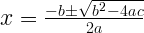Substitution method

This is the most commonly used method in solving simultaneous equation.

Example 1: Let’s solve the pair of equations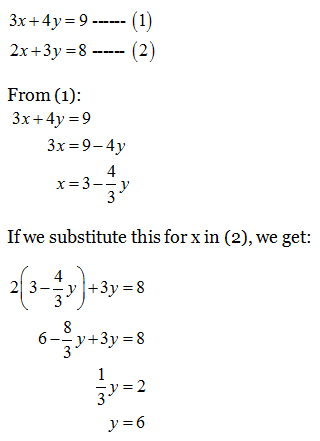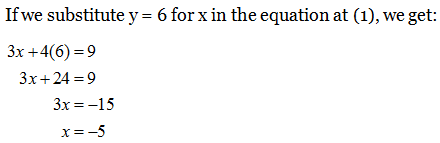We have x = -5, y = 6. As a check, we can substitute both these value in (1) and (2):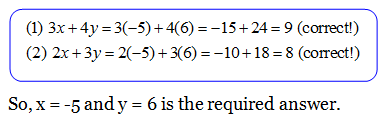Equating coefficients

Example 2: To solve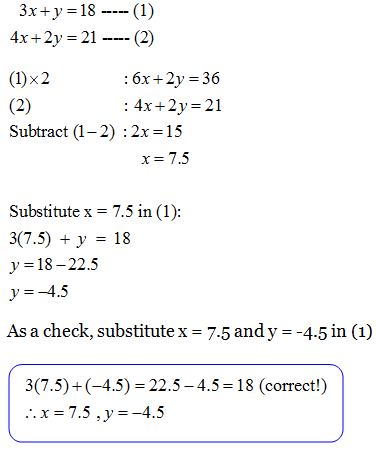So, x = 7.5 and y = -4.5 is the required answer.

Using Formulae

Sometime we can’t use substitution or equating coefficient methods to solve the simultaneous equation, in this case we need to use the formulae:Example 3:

Solve the simultaneous equations$3x+y=2$ and$3{{x}^{2}}+{{y}^{2}}+xy=6$. Give your answers correct to three decimal places.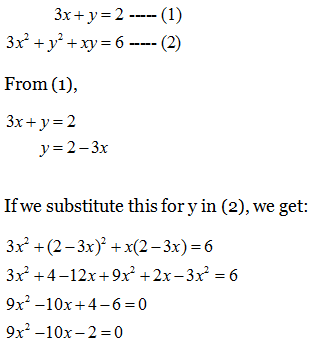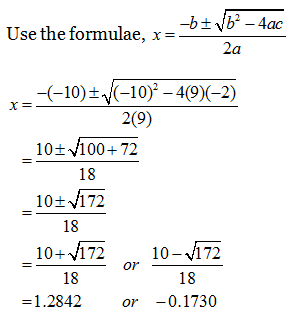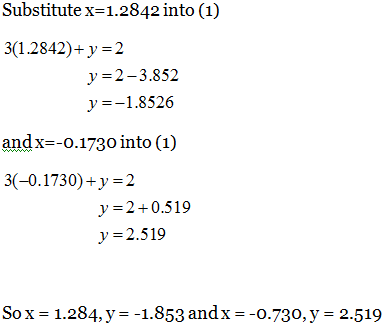Please take note that only round off the final answer correct to 3 decimal places)
You might ask how are we going to know that when we need to use the formulae to solve the simultaneous equations?The little trick is we only need to check the value of$\sqrt{{{b}^{2}}-4ac}$

If the value is a whole number, meant that we can fully factorize the equation.
If the value is the decimal number, then we need to use the formulae.

For example 3 above, after substitution we have the equation of$9{{x}^{2}}-10x-2=0$, let’s calculate its value using$\sqrt{{{b}^{2}}-4ac}$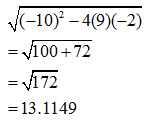We will get a decimal number of 13.1149, hence we need to use formulae to solve the question.

We will look at more SPM questions and example for simultaneous equations in next topic on SPM Questions on Simultaneous Equations.

This site uses Akismet to reduce spam. Learn how your comment data is processed.